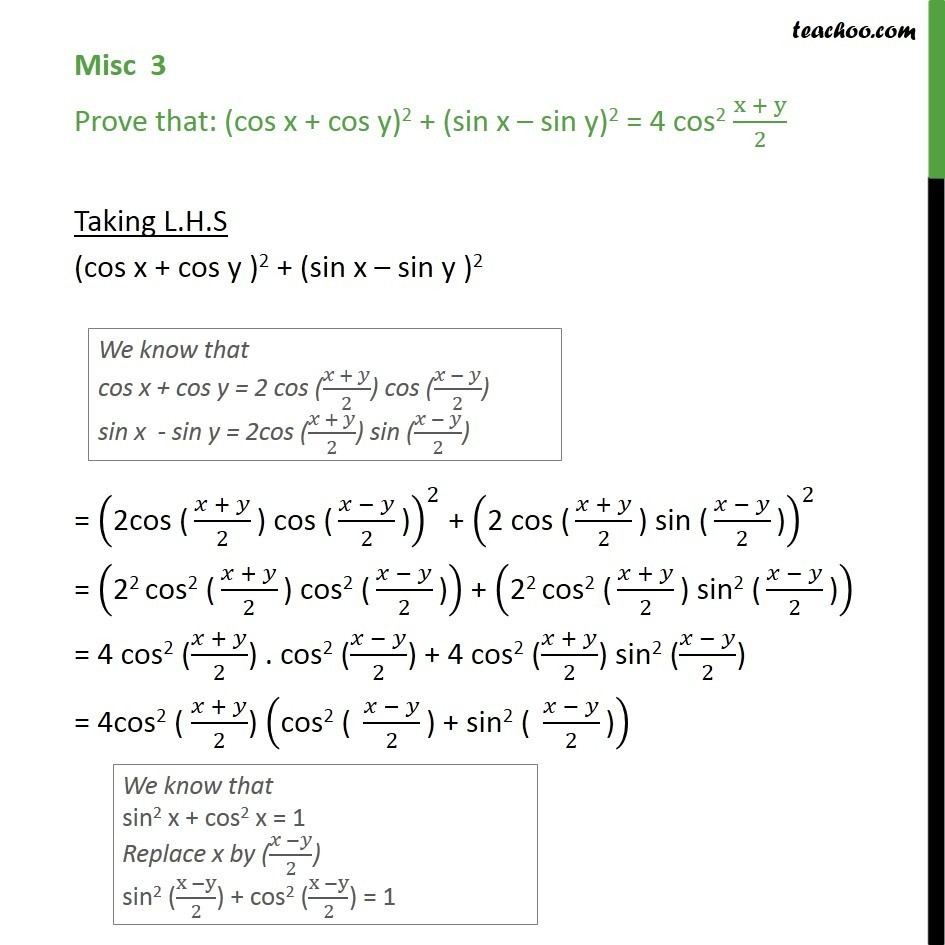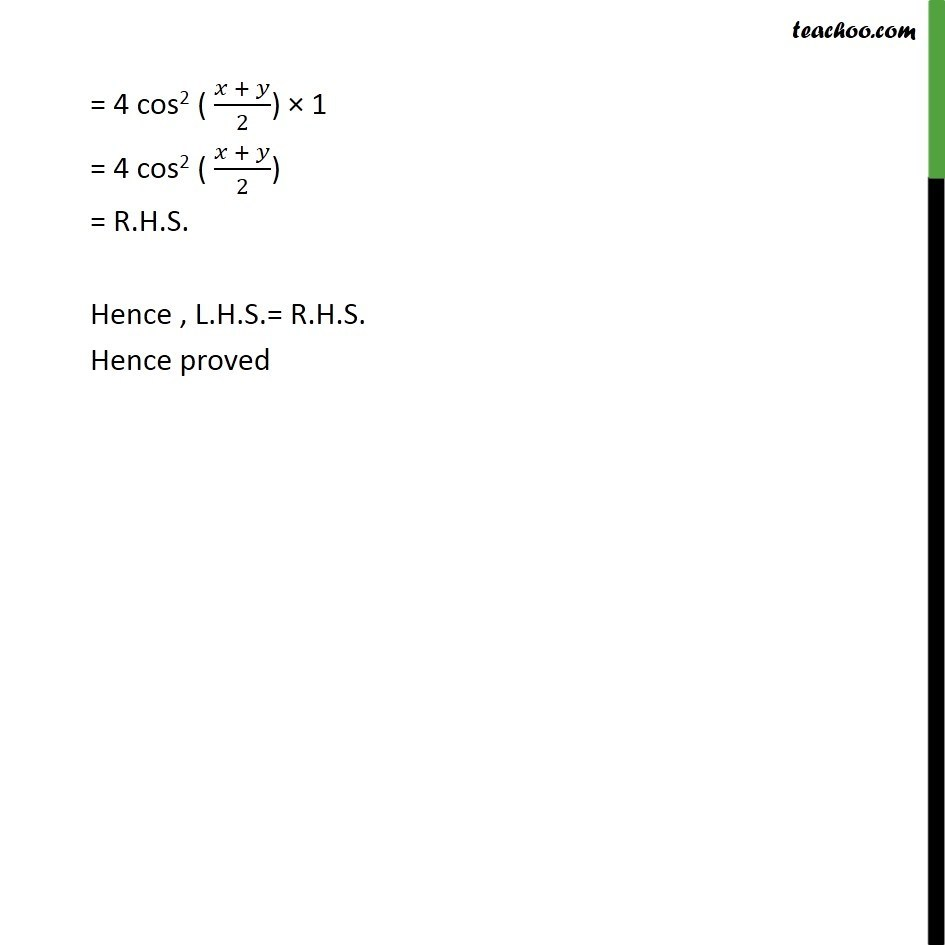1. Chapter 3 Class 11 Trigonometric Functions (Term 2)
2. Serial order wise
3. Miscellaneous

Transcript

Misc 3 Prove that: (cos x + cos y)2 + (sin x – sin y)2 = 4 cos2 (x + y)/2 Taking L.H.S (cos x + cos y )2 + (sin x – sin y )2 = ("2cos (" (𝑥 + 𝑦)/2 ") cos (" (𝑥 − 𝑦)/2 ")" )^2 + ("2 cos (" (𝑥 + 𝑦)/2 ") sin (" (𝑥 − 𝑦)/2 ")" )^2 = ("22 cos2 (" (𝑥 + 𝑦)/2 ") cos2 (" (𝑥 − 𝑦)/2 ")" ) + ("22 cos2 (" (𝑥 + 𝑦)/2 ") sin2 (" (𝑥 − 𝑦)/2 ")" ) = 4 cos2 ((𝑥 + 𝑦)/2) . cos2 ((𝑥 − 𝑦)/2) + 4 cos2 ((𝑥 + 𝑦)/2) sin2 ((𝑥 − 𝑦)/2) = 4cos2 ( (𝑥 + 𝑦)/2) ("cos2 ( " (𝑥 − 𝑦)/2 ") + sin2 ( " (𝑥 − 𝑦)/2 ")" ) = 4 cos2 ( (𝑥 + 𝑦)/2) × 1 = 4 cos2 ( (𝑥 + 𝑦)/2) = R.H.S. Hence , L.H.S.= R.H.S. Hence proved